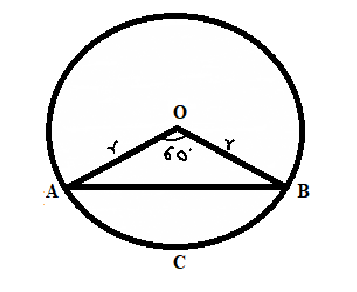#### Find the area of the segment of a circle of radius 12 cm whose corresponding sector has a central angle of 60° (Use = 3.14).

SolutionArea of sector – Area of triangle

Angle = 60°

Area of sector OAB =

DAOB is isosceles triangles

Let

{ Sum of all interior angles of a triangle is 180°}

x + x +60=180

2x =120

x=60

Here all the three angles are 60°        given triangle is an equilateral triangle.

Area of               {  Area of equilateral triangle }

Area of segment = Area of sector OBCA – Area of DAOB

=